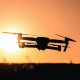## Drone Pilot – Trajectory controller (raspberry pi + naze32)https://youtu.be/k6tswW7M_-8

Impressive right in this video I’m gonna show you how a quadcopter can actually perform this type of trajectory. This is not expulsion. By the way. If you cannot see in my previous videos, I suggest you to watch it because it relates to this one.

The first thing that we’re gonna need is a flight controller. A flight stack in this case were using a nice 3200 Raspberry Pi that they are working together and to come together. In this example, I am actually flying the vehicle manually using Wi-Fi.

This technique is called RC override. The next step is the signing a petition controller that will hold the vehicle in a specified position inside the laboratory. In this case the vehicle is holding 0-0 and 1.

6 meters and regardless the disturbance I make to it. The next step is actually using some math to generate the eggs, my trajectory that the vehicle is going to try to follow it’s, going to try to track.

This is done by using sines and cosines and specifying a time in which the circle is gonna be complete once that we know that the trajectory is is working and under the vehicle can actually perform it.

We perceived to do it experimentally. In this example, you can see the vehicle starts to move in the laboratory and doing the actual circle trajectory this trajectory. It will take around the vehicle around ten seconds now exactly 10 seconds to complete the circle, as you can see here.

But of course, 10 seconds is too slow right. We need, we need to push the envelope and we need to make it faster. So we’re gonna do it at 4 seconds. The vehicle has four seconds to complete an entire circle trajectory.

This is the fastest that this vehicle inside this laboratory can go if we make it faster, we risk of loosing stability, and maybe the vehicle will shake ok, but what about? If you want to do something more interesting and not just a common circle, so we want to do then a figure of a trajectory.

A figure of a trajectory is very common when doing this type of position controllers. If the first thing we have to do is select which figure of eight or infinity trajectory we want to, do I like the one that is a following the red dot, because that that is the one of the day.

Let me skate of Bernoulli, so I choose that one. I did the math for that one and generated the X of Y trajectory, and then I put that trajectory to the raspberry pi and the raspberry PI’s. When I calculate the necessary people.

Roll pitch yaw and total movements for the vehicle to follow that trajectory, but, of course let’s, speed it up. Then again. This is the fastest trajectory that the vehicle can perform inside of this lab and he’s.

Doing the landscape of revenue in six seconds so six seconds it takes to do the entire figure of eight impressive right without pilot. The fact that I can actually do that. We have to consider that this is an automatic pilot.

This is an automatically within MapInfo, and here I is activate the trajectory and the vehicle performance by itself almost series, my vehicle RV turn around. Thank you for watching you